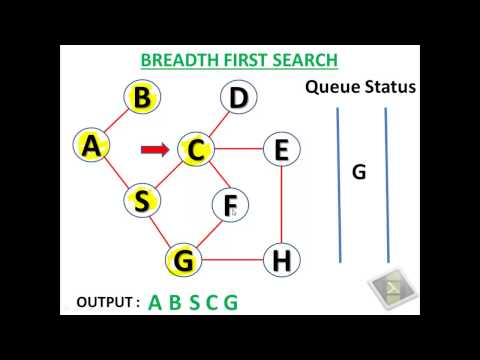# Implementing BFS (Breadth First Search) for Graph Traversal in PythonBFS is one of the basic algorithm for graph traversal. BFS uses a queue to keep track of visited nodes. The basic idea behind the BFS is to visit the adjacent nodes of the starting node, keep them in the queue and mark them as visisted. Then pick up the next node from the queue and continue the process until the queue is empty.

Here is an youtube video which explains the BFS with a nice visual example:BFS is useful to find the shortest path in a graph between two nodes. It can also be used to find out if a graph is connected or not.

Inspite of those benefits, BFS cannot be used for larger graphs because the time complexity is O(# of nodes + # of edges) which gets exponential as the graph size increases.Here is an example implementation of BFS in python:

``````def bfs(graph, start):
visited = []
queue = [start]

while len(queue) > 0:
current = queue.pop(0)
if current in visited:
continue

visited.append(current)
for node in graph[current]:
if node in visited:
continue
queue.append(node)

return visited

if __name__ == '__main__':
graph = {
1: [1, 2, 5],
2: [3, 5],
3: [2, 4],
4: [3, 5, 6],
5: [1, 2, 4],
6: 
}

paths = bfs(graph, 1)

print(paths)
``````

So, now as we learned how to code a BFS, when to use it or it's other counter part DFS? Here is a good discussion about some practical cases regarding when to use BFS or DFS: